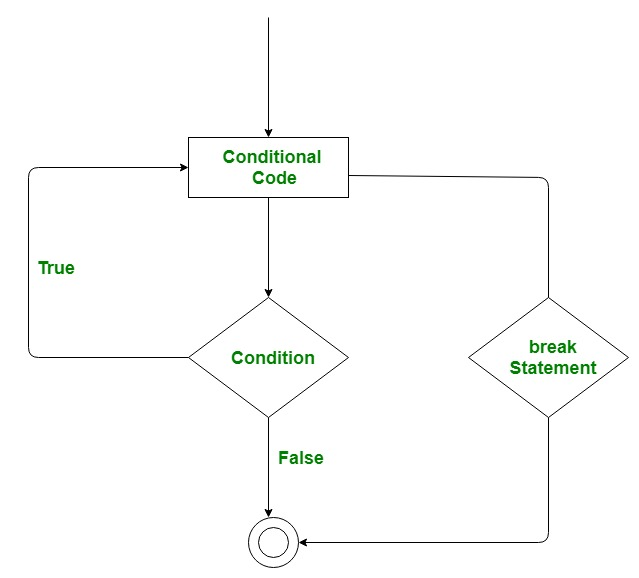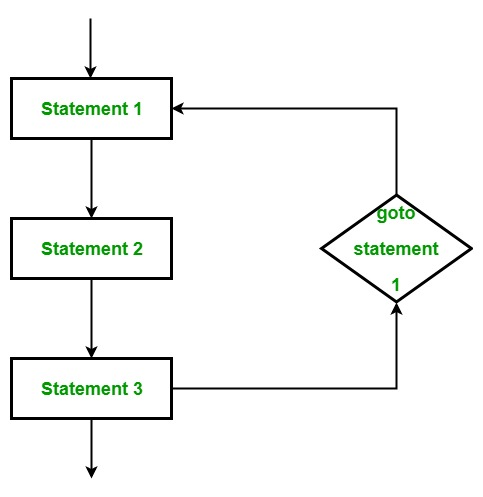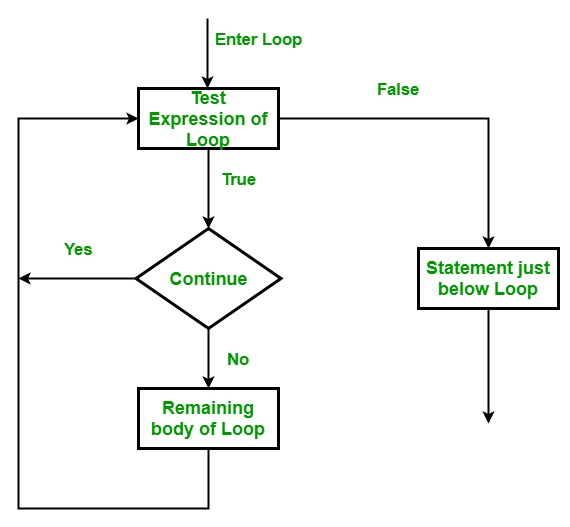# Loop Control Statements in Go Language

• Last Updated : 19 Nov, 2019

Loop control statements in the Go language are used to change the execution of the program. When the execution of the given loop left its scope, then the objects that are created within the scope are also demolished. The Go language supports 3 types of loop control statements:

1. Break
2. Goto
3. Continue

#### Break Statement

The break statement is used to terminate the loop or statement in which it presents. After that, the control will pass to the statements that present after the break statement, if available. If the break statement present in the nested loop, then it terminates only those loops which contains break statement.

Flow Chart:Example:

 `// Go program to illustrate ``// the use of break statement``package main`` ` `import ``"fmt"`` ` `// Main function``func main() {``   ``for` `i:=0; i<5; i++{``        ` `   ``fmt.Println(i)``    ` `   ``// For loop breaks when the value of i = 3``   ``if` `i == 3{``         ``break``;``  ``}``   ``}`` ` `    ` `}`

Output:

```0
1
2
3
```

#### Goto Statement

This statement is used to transfer control to the labeled statement in the program. The label is the valid identifier and placed just before the statement from where the control is transferred. Generally, goto statement is not used by the programmers because it is difficult to trace the control flow of the program.

Flow Chart:Example:

 `// Go program to illustrate ``// the use of goto statement``package main`` ` `import ``"fmt"`` ` `func main() {``   ``var x ``int` `= 0``    ` `   ``// for loop work as a while loop``  ``Lable1: ``for` `x < 8 {``      ``if` `x == 5 {``          ` `         ``// using goto statement``         ``x = x + 1;``         ``goto` `Lable1``      ``}``      ``fmt.Printf(``"value is: %d\n"``, x);``      ``x++;     ``   ``}  ``}`

Output:

```value is: 0
value is: 1
value is: 2
value is: 3
value is: 4
value is: 6
value is: 7
```

#### Continue Statement

This statement is used to skip over the execution part of the loop on a certain condition. After that, it transfers the control to the beginning of the loop. Basically, it skips its following statements and continues with the next iteration of the loop.

Flow Chart:Example:

 `// Go program to illustrate ``// the use of continue statement``package main`` ` `import ``"fmt"`` ` `func main() {``   ``var x ``int` `= 0``    ` `   ``// for loop work as a while loop``   ``for` `x < 8 {``      ``if` `x == 5 {``          ` `         ``// skip two iterations``         ``x = x + 2;``         ``continue``;``      ``}``      ``fmt.Printf(``"value is: %d\n"``, x);``      ``x++;     ``   ``}  ``}`

Output:

```value is: 0
value is: 1
value is: 2
value is: 3
value is: 4
value is: 7
```

My Personal Notes arrow_drop_up